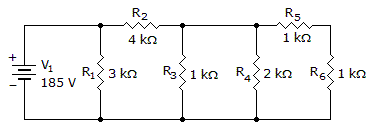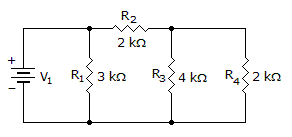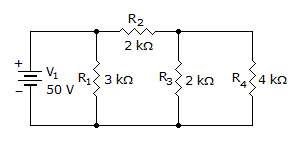# Electronics - Series-Parallel Circuits

### Exercise :: Series-Parallel Circuits - True or False

6.

Bleeder current is the total current left after the total divider current is subtracted from the total current into the circuit.

 A. True B. False

Explanation:

No answer description available for this question. Let us discuss.

7.

When analyzing series–parallel circuit currents, you should start with the current in the branch farthest from the source.

 A. True B. False

Explanation:

No answer description available for this question. Let us discuss.

8.

The power dissipated by R4 is 211 mW.A. True B. False

Explanation:

No answer description available for this question. Let us discuss.

9.

The total resistance in the circuit is 1.6 k.A. True B. False

Explanation:

No answer description available for this question. Let us discuss.

10.

The current through R1 is 17 mA.A. True B. False